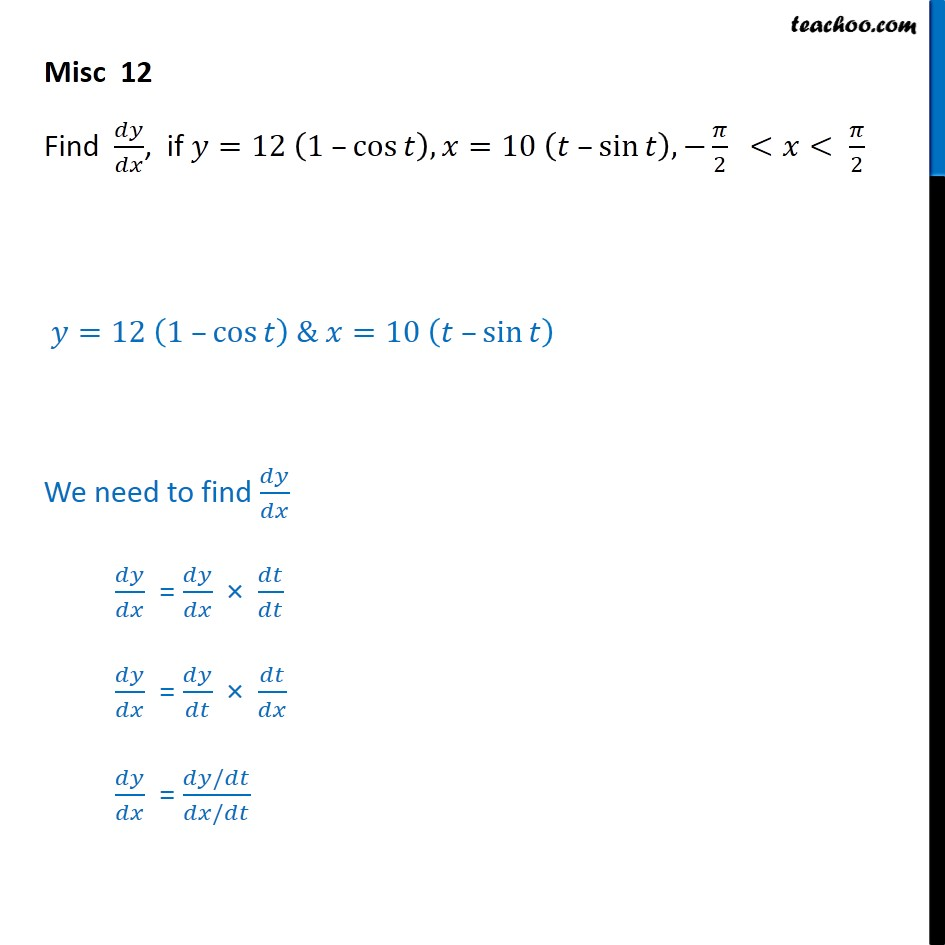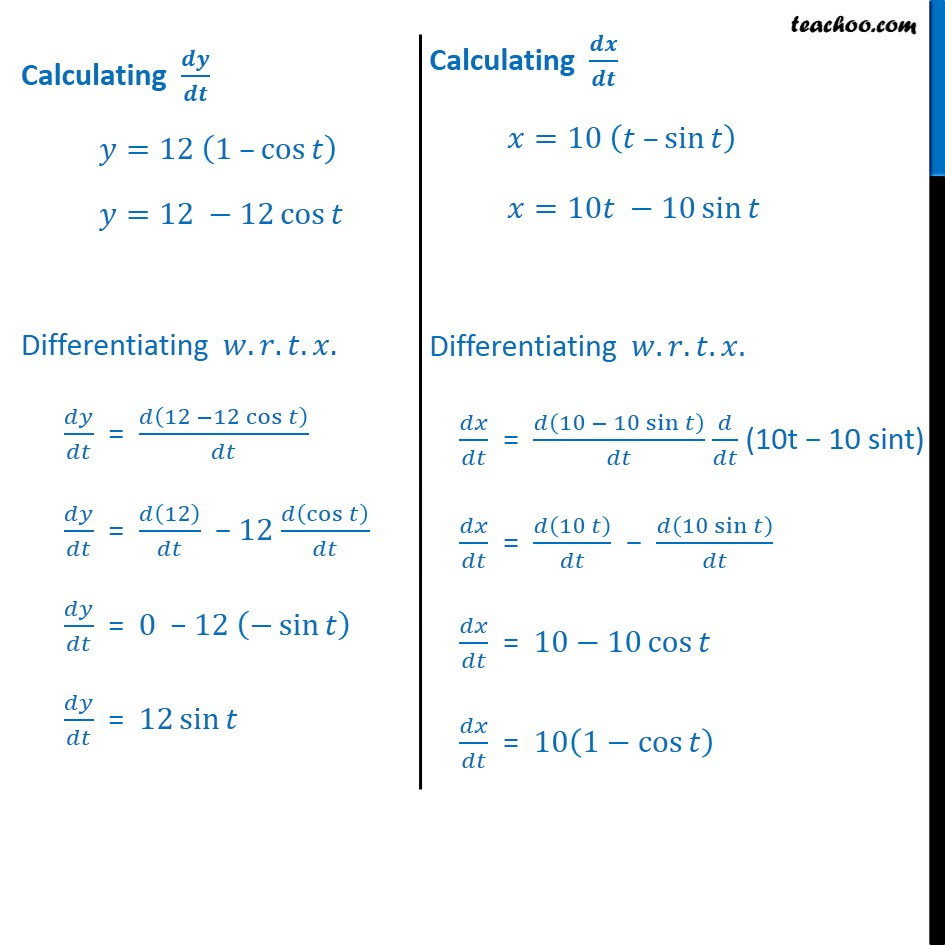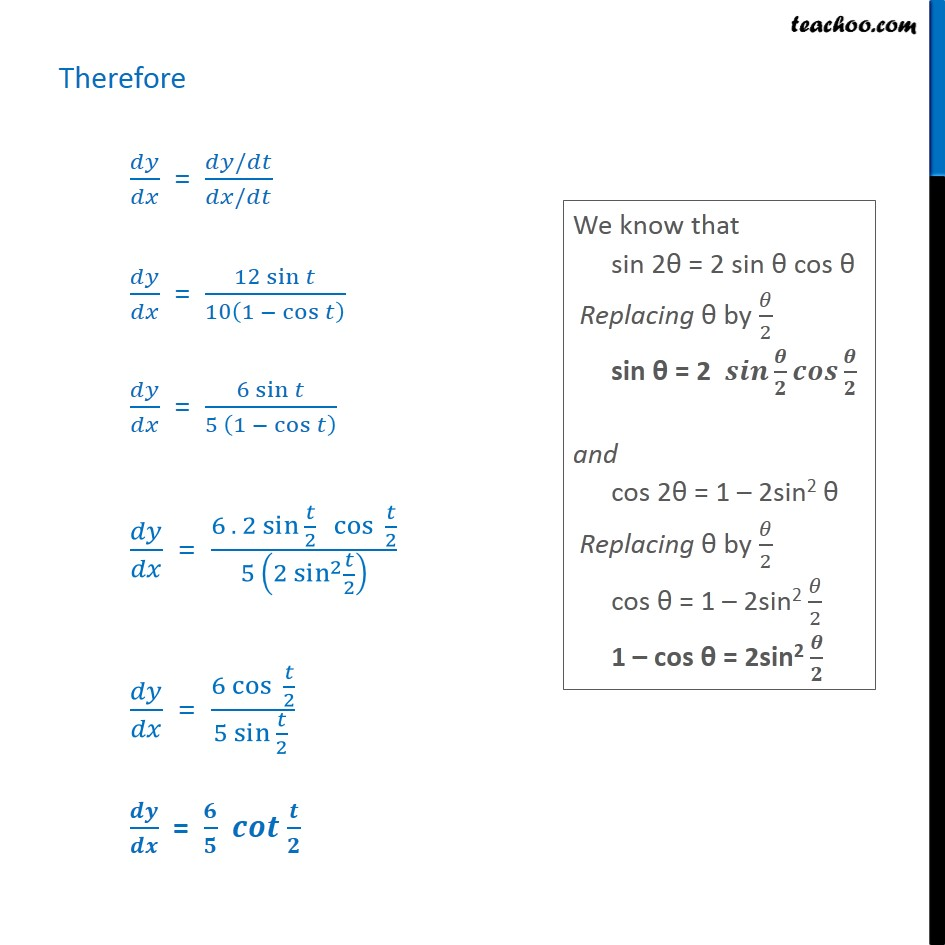Check Full Chapter Explained - Continuity and Differentiability - Continuity and Differentiability Class 121. Chapter 5 Class 12 Continuity and Differentiability
2. Serial order wise
3. Miscellaneous

Transcript

Misc 12 Find , if =12 1 cos , =10 sin , 2 < < 2 =12 1 cos & =10 sin We need to find = = = / / Calculating =12 1 cos =12 12 cos Differentiating . . . . = 12 12 cos = 12 12 cos = 0 12 sin = 12 sin Therefore = / / = 12 sin 10 1 cos = 6 sin 5 1 cos = 6 . 2 sin 2 cos 2 5 2 sin 2 2 = 6 cos 2 5 sin 2 = = 6 2 sin 2 cos 2 5 1 1 2 sin 2 2

Miscellaneous